Home > CC4 > Chapter A > Lesson A.1.7 > ProblemA-72

A-72.For each equation, a possible solution is given. Check to see if the given solution is correct. Homework Help ✎

1. Is x = −4 a solution to 3x + 7 = x − 1?

2. If −2x − 4 = −4x + 3, then does x = 3?

3. Is x = 2 a solution to −3x + 5 + 5x − 1 = 0?

4. If f(x) = −(x − 1) then does f(−2) = 3?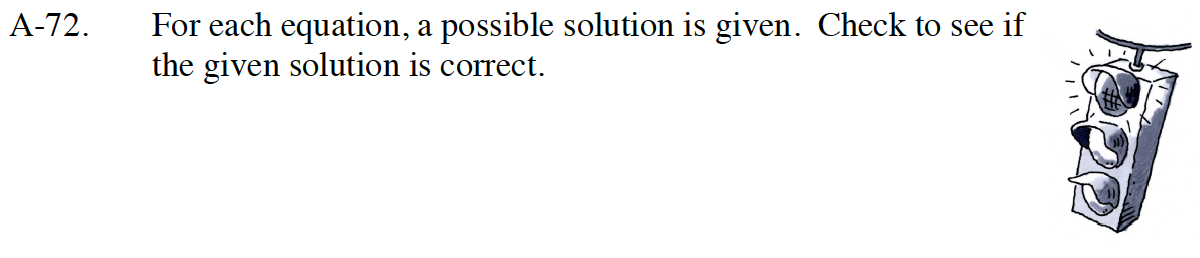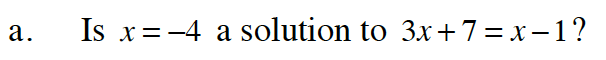Substitute the possible answer in for x.

3( −4 ) + 7 = ( −4 ) − 1

If the numbers on both sides of the equation sign are true, then the possible answer is correct.

−12 + 7 = −5
−5 = −5

Yes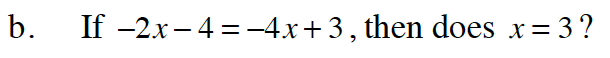Follow the steps in part (a).

No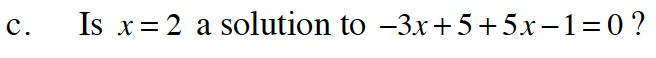Follow the steps in part (a).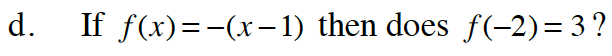How does the negative in front of the parentheses change what is inside?

Follow the steps in part (a).